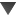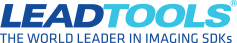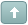Error

#How-To: Create an OCR-Driven Calculator

#1 Posted : Thursday, October 5, 2017 4:40:31 PM(UTC)
This code snippet shows how LEADTOOLS OCR can be used to detect, recognize, and execute simple mathematical statements such as "2 + 2".

Code:
``````
static void SimpleOCRCalculator(string filePath)
{
RasterCodecs codecs = new RasterCodecs();

string[] calculations;
using (IOcrEngine engine = OcrEngineManager.CreateEngine(OcrEngineType.LEAD, false))
{
engine.Startup(null, null, null, null);
IOcrPage page = engine.CreatePage(image, OcrImageSharingMode.None);

page.AutoZone(null);
page.Recognize(null);

calculations = new string[page.Zones.Count];

for (int i = 0; i < page.Zones.Count; i++)
{
calculations[i] = page.GetText(i);
}

engine.Shutdown();
}

Dictionary<string, Action<double, double>> operands = new Dictionary<string, Action<double, double>>();
operands.Add("+", new Action<double, double>(delegate(double a, double b) { double ans = a + b; Console.WriteLine("{0} + {1} = {2}", a, b, ans); }));
operands.Add("-", new Action<double, double>(delegate(double a, double b) { double ans = a - b; Console.WriteLine("{0} - {1} = {2}", a, b, ans); }));
operands.Add("x", new Action<double, double>(delegate(double a, double b) { double ans = a * b; Console.WriteLine("{0} * {1} = {2}", a, b, ans); }));
operands.Add("/", new Action<double, double>(delegate(double a, double b) { double ans = a / b; Console.WriteLine("{0} / {1} = {2}", a, b, ans); }));

for (int i = 0; i < calculations.Length; i++)
{
string equation = Regex.Replace(calculations[i], @"\n|\r| ", "");
string[] ops = new string[] { "+", "-", "x", "/" };

for (int j = 0; j < ops.Length; j++)
{
int index = equation.IndexOf(ops[j]);

if (index > 0 && index < equation.Length)
{
string op1 = equation.Substring(0, index);
string op2 = equation.Substring(index + 1);

double arg1 = double.Parse(op1);
double arg2 = double.Parse(op2);

operands[ops[j]](arg1, arg2);

break;
}
}
}

codecs.Dispose();
image.Dispose();
}
``````

The image used for testing is also included.Edited by user Friday, June 1, 2018 11:53:32 AM(UTC)  | Reason: Update to v20

Nick Crook
Developer Support EngineerTry the latest version of LEADTOOLS for free for 60 days by downloading the evaluation: https://www.leadtools.com/downloadsWanna join the discussion? Login to your LEADTOOLS Support accountor Register a new forum account.